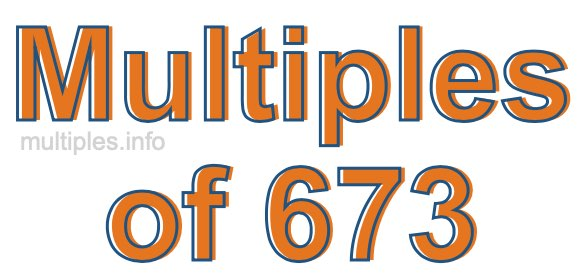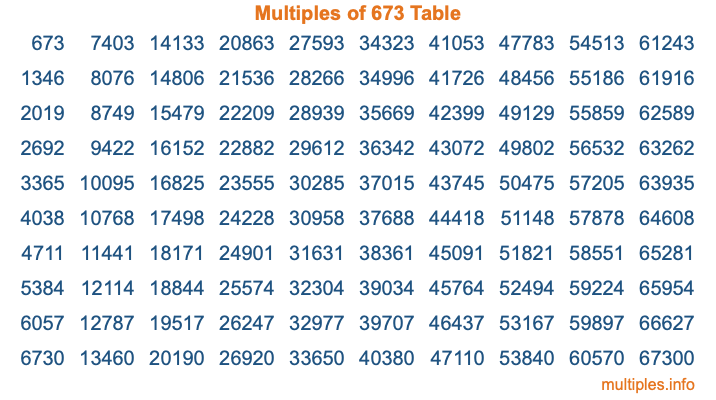Multiples of 673Welcome to the Multiples of 673 page. Here we will first teach you everything you will ever need to know about the multiples of 673, and then give you a study guide summary of everything we taught you to make sure you remember it all. Use this page to look up facts and learn information about the multiples of 673. This page will make you a multiples of six hundred seventy-three expert!

Definition of Multiples of 673
Multiples of 673 are all the numbers that when divided by 673 equal an integer. Each of the multiples of 673 are called a multiple. A multiple of 673 is created by multiplying 673 by an integer.

Therefore, to create a list of multiples of 673, you start with 1 multiplied by 673, then 2 multiplied by 673, then 3 multiplied by 673, and so on for as long as you want. Thus, the list of the first five multiples of 673 is 673, 1346, 2019, 2692, and 3365. To see a larger list of multiples of 673, see the printable image of Multiples of 673 further down on this page. We also have a category where you can choose any nth multiple of 673.

Multiples of 673 Checker
The Multiples of 673 Checker below checks to see if any number of your choice is a multiple of 673. In other words, it checks to see if there is any number (integer) that when multiplied by 673 will equal your number. To do that, we divide your number by 673. If the the quotient is an integer, then your number is a multiple of 673.

Is  a multiple of 673?

Least Common Multiple of 673 and ...
A Least Common Multiple (LCM) is the lowest multiple that two or more numbers have in common. This is also called the smallest common multiple or lowest common multiple and is useful to know when you are adding our subtracting fractions. Enter one or more numbers below (673 is already entered) to find the LCM.

Check out our LCM Calculator if you need more details about the Least Common Multiple or if you need the LCM for different numbers for adding and subtraction fractions.

nth Multiple of 673
As we stated above, 673 is the first multiple of 673, 1346 is the second multiple of 673, 2019 is the third multiple of 673, and so on. Enter a number below to find the nth multiple of 673.

th multiple of 673

Multiples of 673 vs Factors of 673
673 is a multiple of 673 and a factor of 673, but that is where the similarities end. All postive multiples of 673 are 673 or greater than 673. All positive factors of 673 are 673 or less than 673.

Below is the beginning list of multiples of 673 and the factors of 673 so you can compare:

Multiples of 673: 673, 1346, 2019, 2692, 3365, etc.

Factors of 673: 1, 673

As you can see, the multiples of 673 are all the numbers that you can divide by 673 to get a whole number. The factors of 673, on the other hand, are all the whole numbers that you can multiply by another whole number to get 673.

It's also interesting to note that if a number (x) is a factor of 673, then 673 will also be a multiple of that number (x).

Multiples of 673 vs Divisors of 673
The divisors of 673 are all the integers that 673 can be divided by evenly. Below is a list of the divisors of 673.

Divisors of 673: 1, 673

The interesting thing to note here is that if you take any multiple of 673 and divide it by a divisor of 673, you will see that the quotient is an integer.

Multiples of 673 Table
Below is an image of the first 100 multiples of 673 in a table. The table is in chronological order, column by column. The first column has the first ten multiples of 673, the second column has the next ten multiples of 673, and so on.The Multiples of 673 Table is also referred to as the 673 Times Table or Times Table of 673. You are welcome to print out our table for your studies.

Negative Multiples of 673
Although not often discussed or needed in math, it is worth mentioning that you can make a list of negative multiples of 673 by multiplying 673 by -1, then by -2, then by -3, and so on, to get the following list of negative multiples of 673:

-673, -1346, -2019, -2692, -3365, etc.

Multiples of 673 Summary
Below is a summary of important Multiples of 673 facts that we have discussed on this page. To retain the knowledge on this page, we recommend that you read through the summary and explain to yourself or a study partner why they hold true.

There are an infinite number of multiples of 673.

A multiple of 673 divided by 673 will equal a whole number.

673 divided by a factor of 673 equals a divisor of 673.

The nth multiple of 673 is n times 673.

The largest factor of 673 is equal to the first positive multiple of 673.

673 is a multiple of every factor of 673.

673 is a multiple of 673.

A multiple of 673 divided by a divisor of 673 equals an integer.

673 divided by a divisor of 673 equals a factor of 673.

Any integer times 673 will equal a multiple of 673.

Multiples of a Number
Here you can get the multiples of another number, all with the same attention to detail as we did for multiples of 673 on this page.

Multiples of
Multiples of 674
Did you find our page about multiples of six hundred seventy-three educational? Do you want more knowledge? Check out the multiples of the next number on our list!